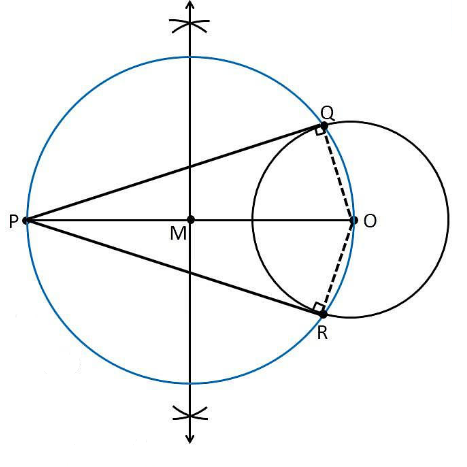Guru

# Draw a circle of radius 6 cm. From a point 10 cm away from its centre, construct the pair of tangents to the circle and measure their lengths. Q.1

• 0

What is the best way to solve the problem of question from Construction ncert class 10th math chapter of question no.1, give me the best and the simple way to solve this problem Draw a circle of radius 6 cm. From a point 10 cm away from its centre, construct the pair of tangents to the circle and measure their lengths.

Share

1. Construction Procedure:

The construction to draw a pair of tangents to the given circle is as follows.

1. Draw a circle with radius = 6 cm with centre O.

2. Locate a point P, which is 10 cm away from O.

3. Join the points O and P through line

4. Draw the perpendicular bisector of the line OP.

5. Let M be the mid-point of the line PO.

6. Take M as centre and measure the length of MO

7. The length MO is taken as radius and draw a circle.

8. The circle drawn with the radius of MO, intersect the previous circle at point Q and R.

9. Join PQ and PR.

10. Therefore, PQ and PR are the required tangents.Justification:

The construction of the given problem can be justified by proving that PQ and PR are the tangents to the circle of radius 6cm with centre O.

To prove this, join OQ and OR represented in dotted lines.

From the construction,

∠PQO is an angle in the semi-circle.

We know that angle in a semi-circle is a right angle, so it becomes,

∴ ∠PQO = 90°

Such that

⇒ OQ ⊥ PQ

Since OQ is the radius of the circle with radius 6 cm, PQ must be a tangent of the circle. Similarly, we can prove that PR is a tangent of the circle.

Hence, justified.

• 0